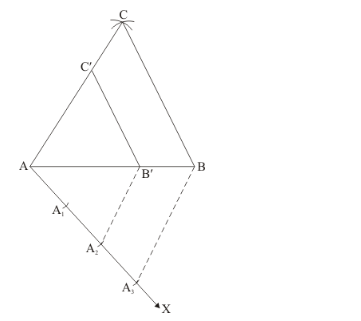# Construct a triangle of sides 4 cm, 5 cm and 6 cm and then a triangle

Question:

Construct a triangle of sides 4 cm, 5 cm and 6 cm and then a triangle similar to it whose sides are (2/3)  of the corresponding sides of it.

Solution:

Given that

Construct a triangle of sides $4 \mathrm{~cm}, 5 \mathrm{~cm}$ and $6 \mathrm{~cm}$ and then a triangle similar to it whose sides are $(2 / 3)$ of the corresponding sides of it.

We follow the following steps to construct the givenStep of construction

Step: I- First of all we draw a line segment.

Step: II- With as centre and radius, draw an arc.

Step: III- With as centre and radius, draw an arc, intersecting the arc drawn in step II at C.

Step: IV- Joins AC and BC to obtain.

Step: V- Below AB, makes an acute angle.

Step: VI- Along AX, mark off three pointssuch thatStep: VII- Join.

Step: VIII- Since we have to construct a triangle each of whose sides is two-third of the corresponding sides of.

So, we take two parts out of three equal parts on AX from pointdrawand meeting AB at C’.

Step: IX- From B’ drawand meeting AC at C’

Thus,is the required triangle, each of whose sides is two third of the corresponding sides of.